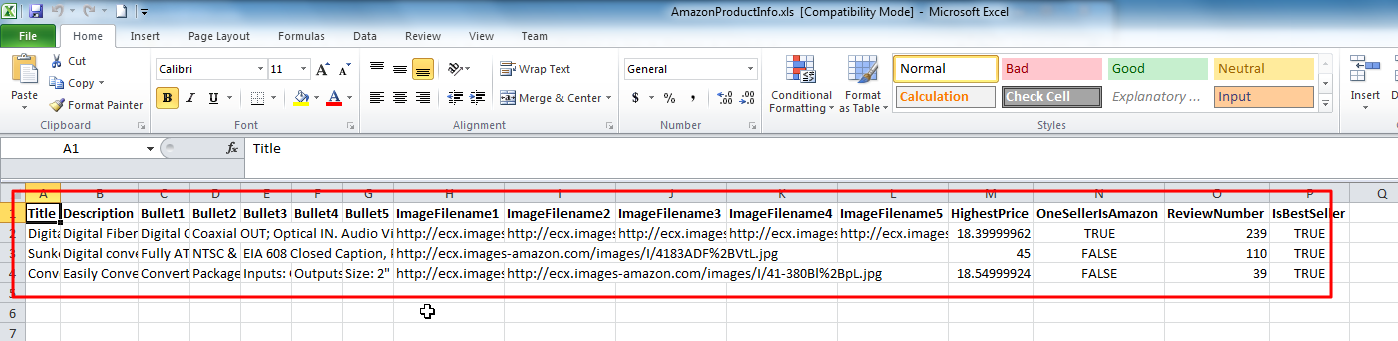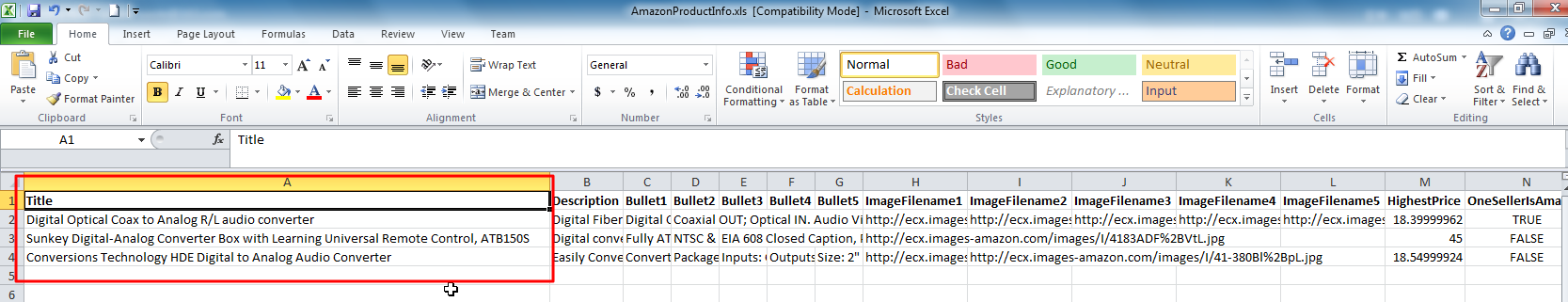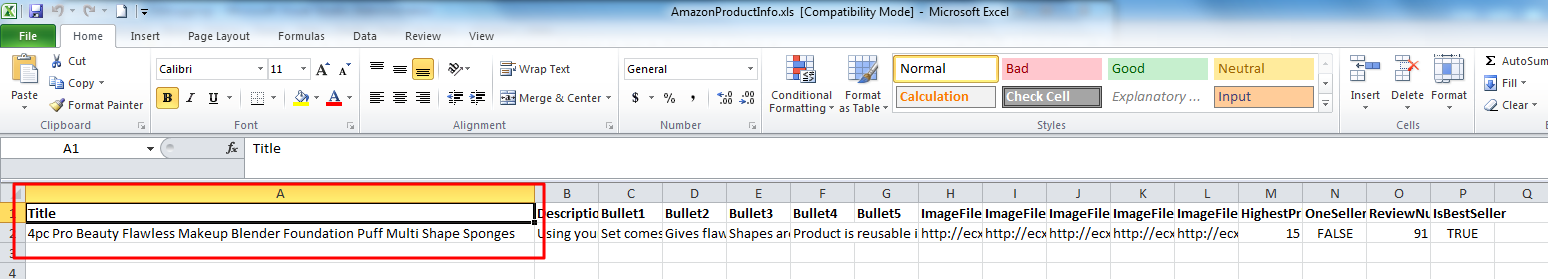# 【已解决】C#中操作Excel文件实现单行的自动适应列宽+C#中如何选中Excel的某列

C# 5508浏览

【背景】

【已解决】C#中操作刚导出的Excel，设置其为自动调整列宽【折腾过程】

1.之前的，全部都自动适应列宽的代码是：

```            //(2) auto adjust column width (according to content)
if (isAutoFit)
{
Range allColumn = xlWorkSheet.Columns;
allColumn.AutoFit();
}```

2.看起来，貌似可以直接通过：

```            bool isAutoFitForFistColumn = true;
if (isAutoFitForFistColumn)
{
Range firstColumn = (Range)xlWorkSheet.Columns;
firstColumn.AutoFit();
}```

`Range firstColumn = (Range)xlWorkSheet.Columns;`

3.问题转化为了：

C#中如何选中Excel的某列

http://bbs.csdn.net/topics/50481843

```                Range firstColumn = xlWorkSheet.get_Range("A1");
//Range firstColumn = (Range)xlWorkSheet.Columns;
firstColumn.AutoFit();```

`firstColumn.AutoFit();`

4.后来参考：

C# Excel列宽自适应

```                Range firstColumn = xlWorkSheet.get_Range("A1");
//Range firstColumn = (Range)xlWorkSheet.Columns;
firstColumn.EntireColumn.AutoFit();```【总结】

```Range firstColumn = xlWorkSheet.get_Range("A1");
firstColumn.EntireColumn.AutoFit();```

56 queries in 0.119 seconds, using 18.59MB memory